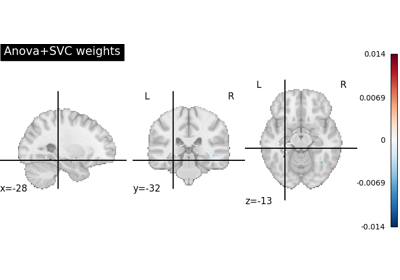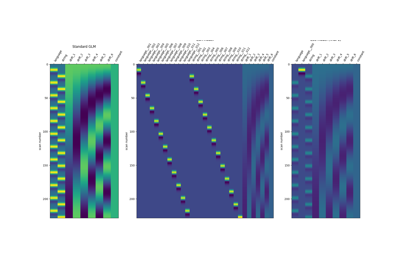Note

This page is a reference documentation. It only explains the function signature, and not how to use it. Please refer to the user guide for the big picture.

# nilearn.plotting.plot_stat_map#

nilearn.plotting.plot_stat_map(stat_map_img, bg_img=<MNI152Template>, cut_coords=None, output_file=None, display_mode='ortho', colorbar=True, cbar_tick_format='%.2g', figure=None, axes=None, title=None, threshold=1e-06, annotate=True, draw_cross=True, black_bg='auto', cmap=<matplotlib.colors.LinearSegmentedColormap object>, symmetric_cbar='auto', dim='auto', vmax=None, resampling_interpolation='continuous', **kwargs)[source]#

Plot cuts of an ROI/mask image (by default 3 cuts: Frontal, Axial, and Lateral)

Parameters:
stat_map_imgNiimg-like object

See Input and output: neuroimaging data representation. The statistical map image

bg_imgNiimg-like object, optional

See Input and output: neuroimaging data representation. The background image to plot on top of. If nothing is specified, the MNI152 template will be used. To turn off background image, just pass “bg_img=None”. Default=MNI152TEMPLATE.

cut_coordsNone, a `tuple` of `float`, or `int`, optional

The MNI coordinates of the point where the cut is performed.

• If `display_mode` is ‘ortho’ or ‘tiled’, this should be a 3-tuple: `(x, y, z)`

• For `display_mode == 'x'`, ‘y’, or ‘z’, then these are the coordinates of each cut in the corresponding direction.

• If `None` is given, the cuts are calculated automatically.

• If `display_mode` is ‘mosaic’, and the number of cuts is the same for all directions, `cut_coords` can be specified as an integer. It can also be a length 3 tuple specifying the number of cuts for every direction if these are different.

Note

If `display_mode` is ‘x’, ‘y’ or ‘z’, `cut_coords` can be an integer, in which case it specifies the number of cuts to perform.

output_file`str`, or None, optional

The name of an image file to export the plot to. Valid extensions are .png, .pdf, .svg. If `output_file` is not None, the plot is saved to a file, and the display is closed.

display_mode{‘ortho’, ‘tiled’, ‘mosaic’,’x’,’y’, ‘z’, ‘yx’, ‘xz’, ‘yz’}, optional

Choose the direction of the cuts:

• ‘x’: sagittal

• ‘y’: coronal

• ‘z’: axial

• ‘ortho’: three cuts are performed in orthogonal directions

• ‘tiled’: three cuts are performed and arranged in a 2x2 grid

• ‘mosaic’: three cuts are performed along multiple rows and columns

Default=’ortho’.

colorbar`bool`, optional

If `True`, display a colorbar on the right of the plots. Default=True.

cbar_tick_format: str, optional

Controls how to format the tick labels of the colorbar. Ex: use “%i” to display as integers. Default is ‘%.2g’ for scientific notation.

figure`int`, or `matplotlib.figure.Figure`, or None, optional

Matplotlib figure used or its number. If `None` is given, a new figure is created.

axes`matplotlib.axes.Axes`, or 4 tupleof `float`: (xmin, ymin, width, height), optional

The axes, or the coordinates, in matplotlib figure space, of the axes used to display the plot. If `None`, the complete figure is used.

title`str`, or None, optional

The title displayed on the figure. Default=None.

thresholda number, None, or ‘auto’, optional

If `None` is given, the image is not thresholded. If a number is given, it is used to threshold the image: values below the threshold (in absolute value) are plotted as transparent. If ‘auto’ is given, the threshold is determined magically by analysis of the image. Default=1e-6.

annotate`bool`, optional

If `annotate` is `True`, positions and left/right annotation are added to the plot. Default=True.

draw_cross`bool`, optional

If `draw_cross` is `True`, a cross is drawn on the plot to indicate the cut position. Default=True.

black_bg`bool`, or ‘auto’, optional

If `True`, the background of the image is set to be black. If you wish to save figures with a black background, you will need to pass facecolor=’k’, edgecolor=’k’ to `matplotlib.pyplot.savefig`. Default=’auto’.

cmap`matplotlib.colors.Colormap`, or `str`, optional

The colormap to use. Either a string which is a name of a matplotlib colormap, or a matplotlib colormap object.

Note

The colormap must be symmetrical.

Default=`plt.cm.cold_hot`.

symmetric_cbar`bool`, or ‘auto’, optional

Specifies whether the colorbar should range from `-vmax` to `vmax` or from `vmin` to `vmax`. Setting to ‘auto’ will select the latter if the range of the whole image is either positive or negative.

Note

The colormap will always range from `-vmax` to `vmax`.

Default=’auto’.

dim`float`, or ‘auto’, optional

Dimming factor applied to background image. By default, automatic heuristics are applied based upon the background image intensity. Accepted float values, where a typical span is between -2 and 2 (-2 = increase contrast; 2 = decrease contrast), but larger values can be used for a more pronounced effect. 0 means no dimming. Default=’auto’.

vmax`float`, optional

Upper bound of the colormap. If `None`, the max of the image is used. Passed to `matplotlib.pyplot.imshow`.

resampling_interpolation`str`, optional

Interpolation to use when resampling the image to the destination space. Can be:

• “continuous”: use 3rd-order spline interpolation

• “nearest”: use nearest-neighbor mapping.

Note

“nearest” is faster but can be noisier in some cases.

Default=’continuous’.

`nilearn.plotting.plot_anat`

To simply plot anatomical images

`nilearn.plotting.plot_epi`

To simply plot raw EPI images

`nilearn.plotting.plot_glass_brain`

To plot maps in a glass brain

Notes

Arrays should be passed in numpy convention: (x, y, z) ordered.

For visualization, non-finite values found in passed ‘stat_map_img’ or ‘bg_img’ are set to zero.

## Examples using `nilearn.plotting.plot_stat_map`#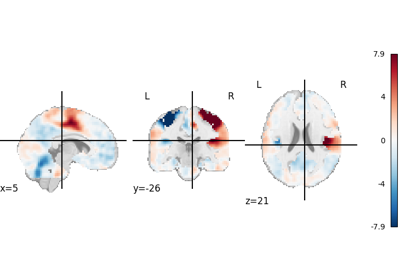3D and 4D niimgs: handling and visualizing

3D and 4D niimgs: handling and visualizing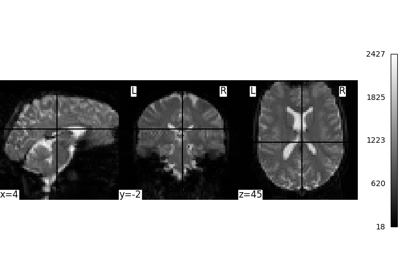Intro to GLM Analysis: a single-session, single-subject fMRI dataset

Intro to GLM Analysis: a single-session, single-subject fMRI dataset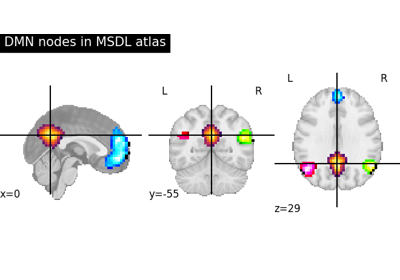Visualizing a probabilistic atlas: the default mode in the MSDL atlas

Visualizing a probabilistic atlas: the default mode in the MSDL atlas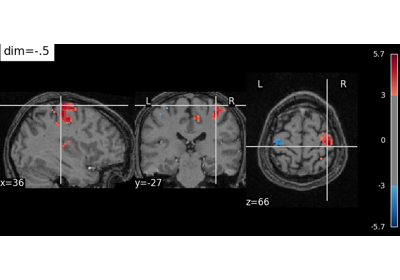Controlling the contrast of the background when plotting

Controlling the contrast of the background when plotting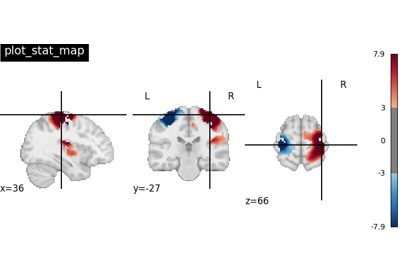Plotting tools in nilearn

Plotting tools in nilearn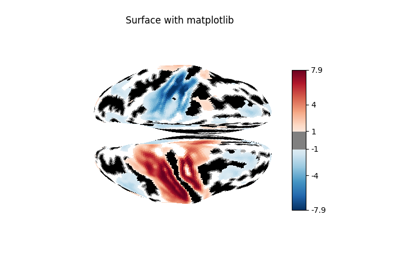Making a surface plot of a 3D statistical map

Making a surface plot of a 3D statistical map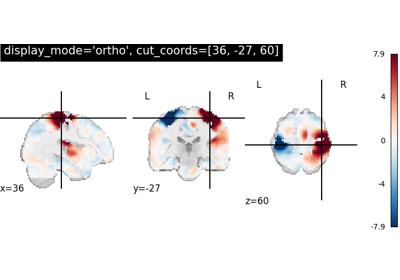More plotting tools from nilearn

More plotting tools from nilearn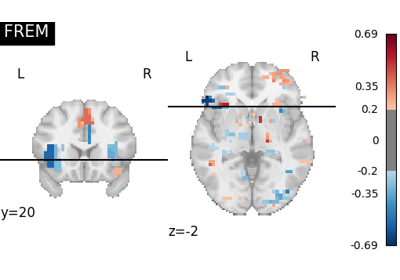FREM on Jimura et al “mixed gambles” dataset

FREM on Jimura et al "mixed gambles" dataset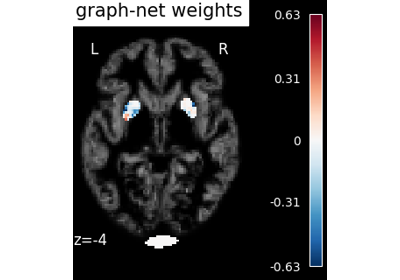Voxel-Based Morphometry on Oasis dataset with Space-Net prior

Voxel-Based Morphometry on Oasis dataset with Space-Net prior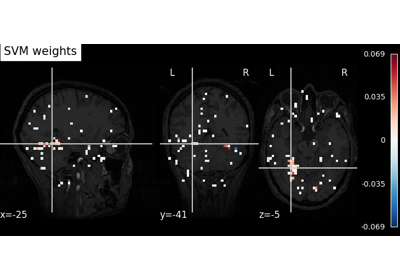Decoding with ANOVA + SVM: face vs house in the Haxby dataset

Decoding with ANOVA + SVM: face vs house in the Haxby dataset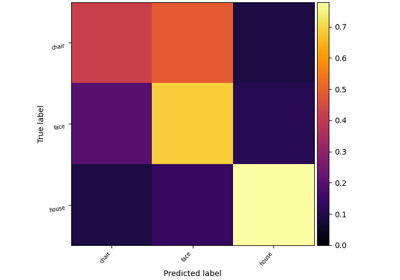Decoding with FREM: face vs house vs chair object recognition

Decoding with FREM: face vs house vs chair object recognition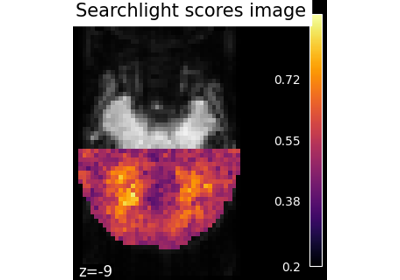Searchlight analysis of face vs house recognition

Searchlight analysis of face vs house recognition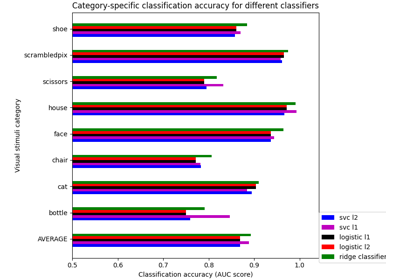Different classifiers in decoding the Haxby dataset

Different classifiers in decoding the Haxby dataset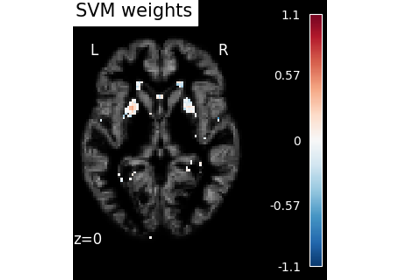Voxel-Based Morphometry on Oasis dataset

Voxel-Based Morphometry on Oasis dataset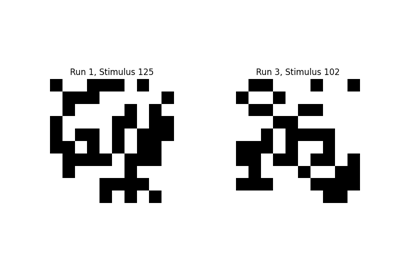Encoding models for visual stimuli from Miyawaki et al. 2008

Encoding models for visual stimuli from Miyawaki et al. 2008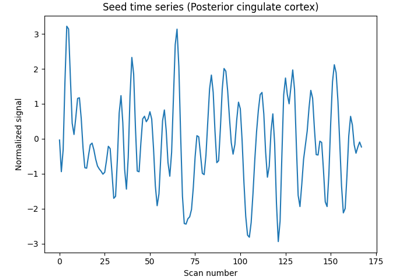Producing single subject maps of seed-to-voxel correlation

Producing single subject maps of seed-to-voxel correlation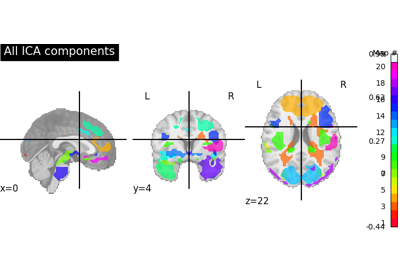Deriving spatial maps from group fMRI data using ICA and Dictionary Learning

Deriving spatial maps from group fMRI data using ICA and Dictionary Learning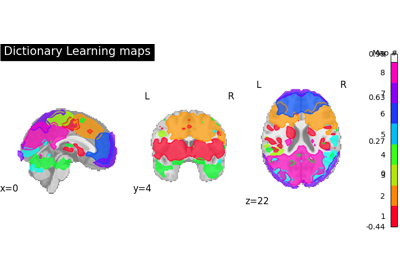Regions extraction using dictionary learning and functional connectomes

Regions extraction using dictionary learning and functional connectomes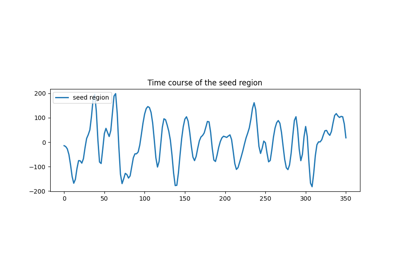Default Mode Network extraction of ADHD dataset

Default Mode Network extraction of ADHD datasetExample of explicit fixed effects fMRI model fitting

Example of explicit fixed effects fMRI model fitting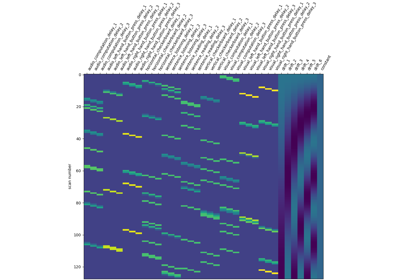Analysis of an fMRI dataset with a Finite Impule Response (FIR) model

Analysis of an fMRI dataset with a Finite Impule Response (FIR) model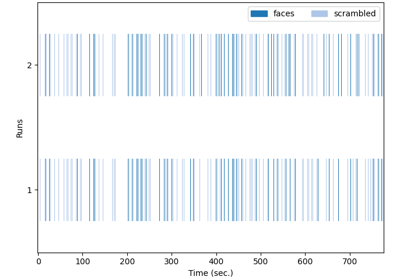Single-subject data (two sessions) in native space

Single-subject data (two sessions) in native spaceSimple example of two-session fMRI model fitting

Simple example of two-session fMRI model fitting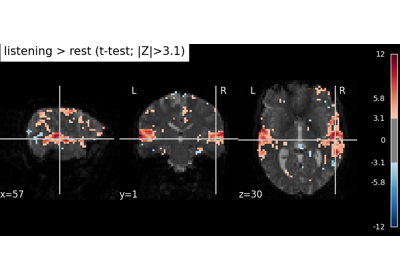Predicted time series and residuals

Predicted time series and residuals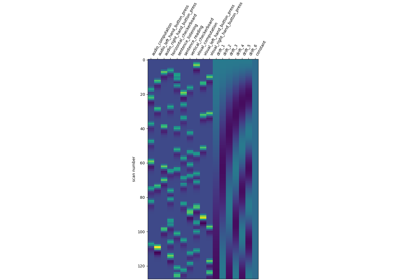Understanding parameters of the first-level model

Understanding parameters of the first-level model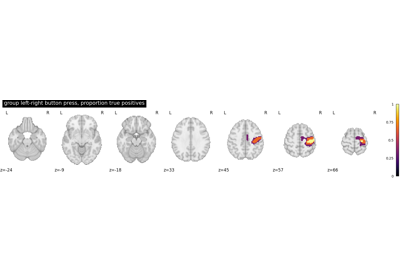Second-level fMRI model: true positive proportion in clusters

Second-level fMRI model: true positive proportion in clusters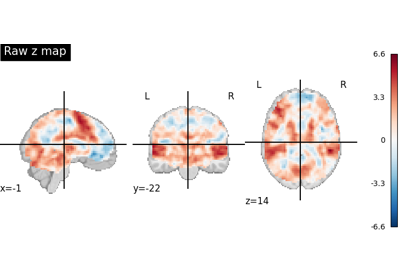Statistical testing of a second-level analysis

Statistical testing of a second-level analysis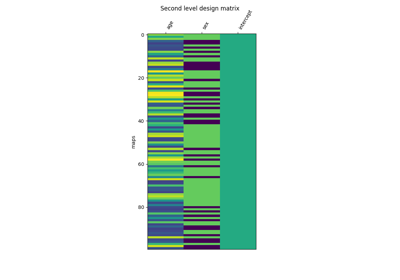Voxel-Based Morphometry on OASIS dataset

Voxel-Based Morphometry on OASIS dataset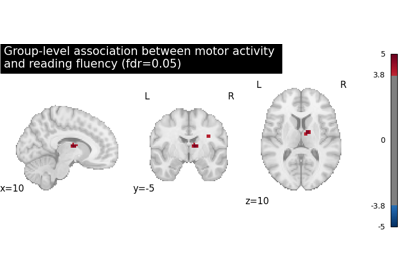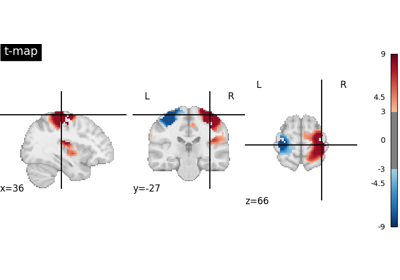Negating an image with math_img

Negating an image with math_img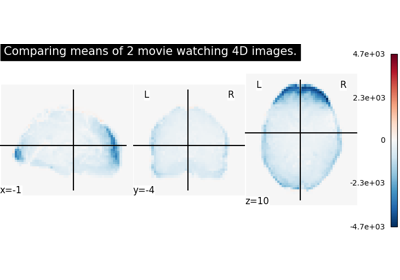Comparing the means of 2 images

Comparing the means of 2 images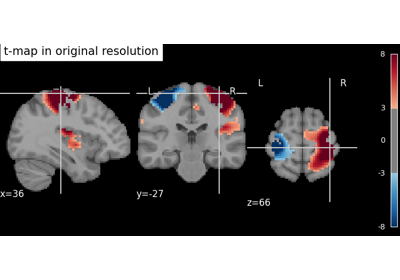Resample an image to a template

Resample an image to a template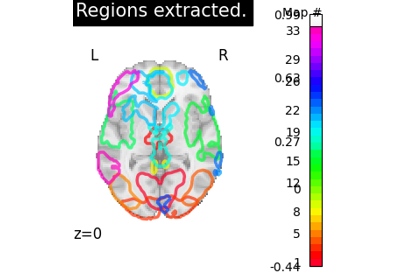Regions Extraction of Default Mode Networks using Smith Atlas

Regions Extraction of Default Mode Networks using Smith Atlas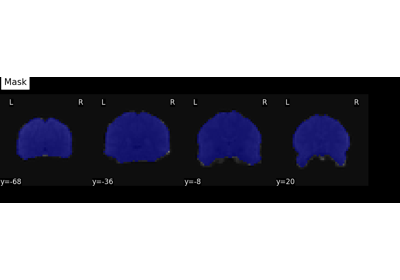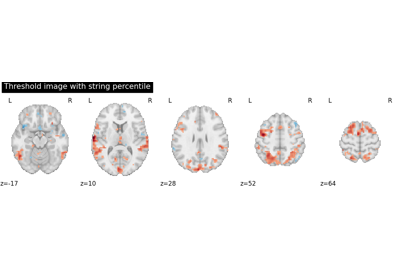Region Extraction using a t-statistical map (3D)

Region Extraction using a t-statistical map (3D)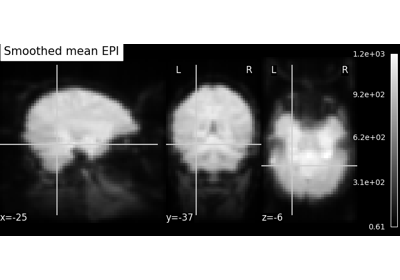Computing a Region of Interest (ROI) mask manually

Computing a Region of Interest (ROI) mask manually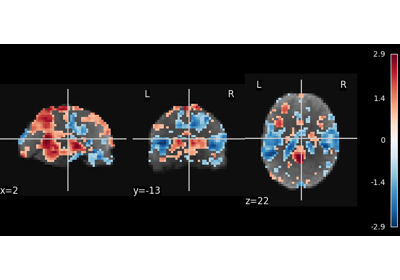Multivariate decompositions: Independent component analysis of fMRI

Multivariate decompositions: Independent component analysis of fMRI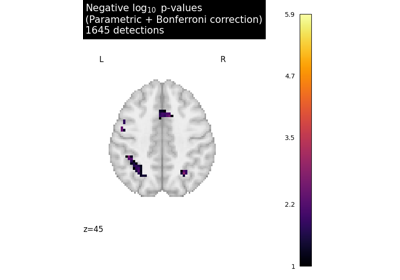Massively univariate analysis of a calculation task from the Localizer dataset

Massively univariate analysis of a calculation task from the Localizer dataset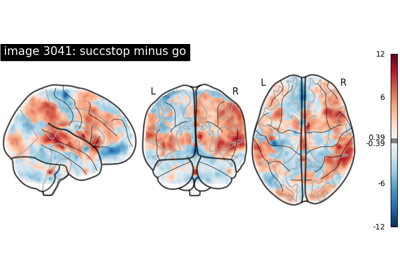NeuroVault meta-analysis of stop-go paradigm studies

NeuroVault meta-analysis of stop-go paradigm studies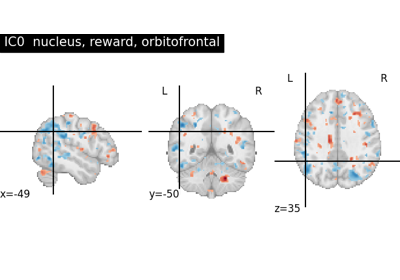NeuroVault cross-study ICA maps

NeuroVault cross-study ICA maps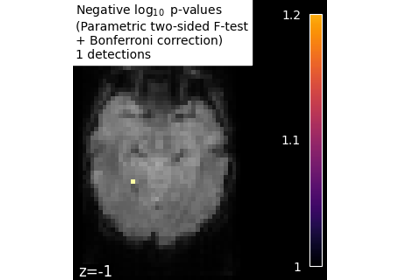Massively univariate analysis of face vs house recognition

Massively univariate analysis of face vs house recognition